## Human-Motion-Analysis

버전 0.0.1 (20.1 MB) 작성자:
Human motion capture system

다운로드 수: 43

업데이트 날짜: 2022/3/18

GitHub에서 호스트

# Human Motion Analysis Programming

Supervised by Prof. Tung-Wu Lu, Dept. of BioMedical Engineering, NTU

MATLAB programs, which use the data derived from a motion capture system and force plates, for human motion analysis, including motion tracking, evaluation of body balance, and derivation of joint forces and torques of the lower body, etc.

## Requirements

1. MATLAB 2015 (or newer versions)
2. MTIMESX function(Fast Matrix Multiply with Multi-Dimensional)

## Functions & Execution Results

### Week1: Transformation between Global & Local Coordinate

The transformation between global & local coordinate of marker position on lower body segemnts.

### Week2: COP Tracking

Derive the COP from data of two force plates and display the positions relative to force plates.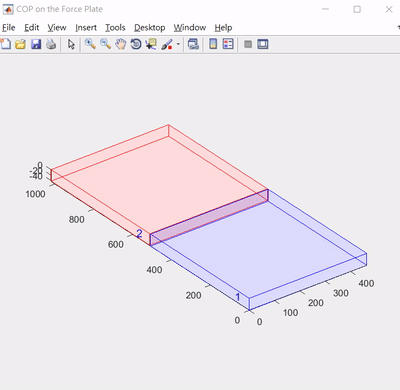### Week3: Euler Angle & Fixed Angle

1. Represent the rotations of body segemnts with Euler angle and fixed angle.
2. Display the difference of Euler angle before and after static calibration of indivisual.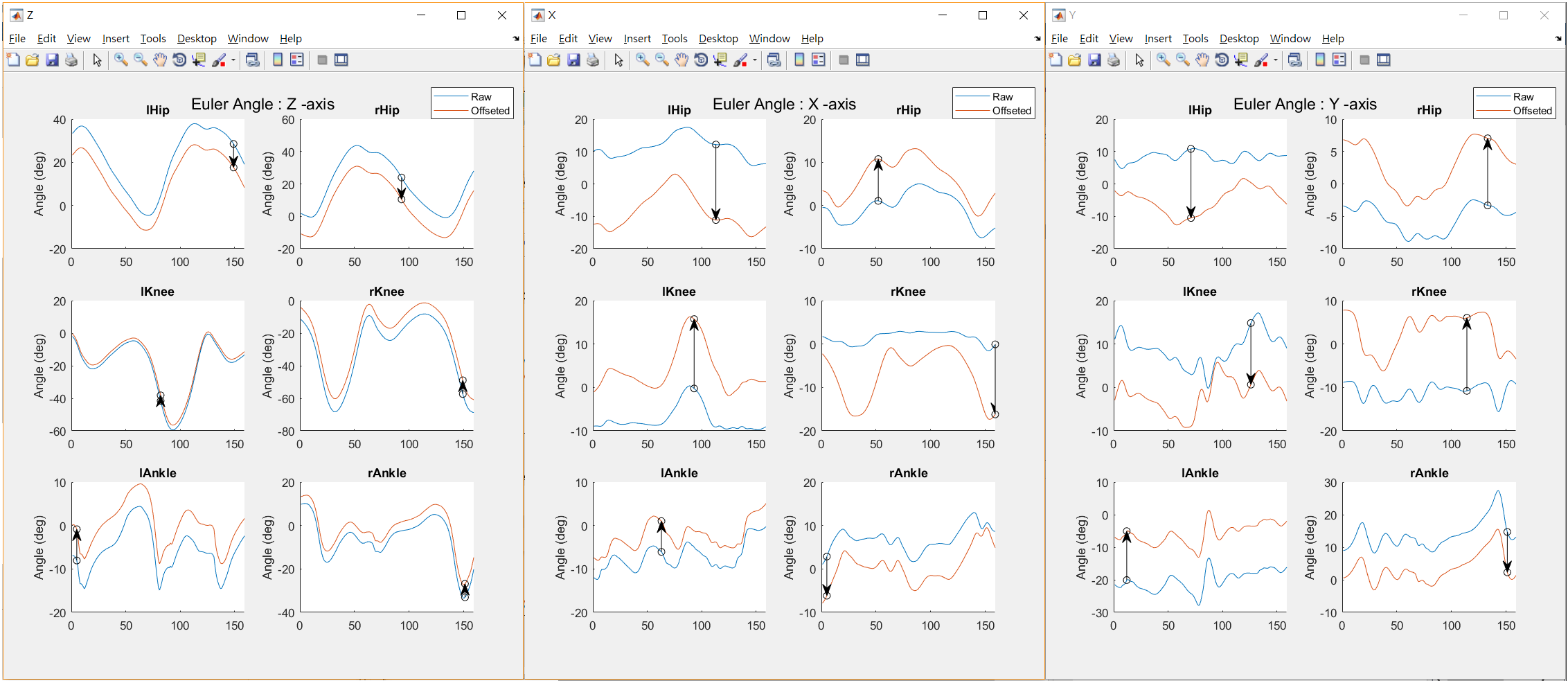### Week4: Curve Fitting

1. Smoothen the data curve and display the angular velocity of lower body.
2. Consider right foot only, compare the results of analytic solution of angular acceleration & 1st derivation of angular velocity.
3. Consider right thigh only, compare the angular velocity derive from 12 sequence of Euler angle.
The one with Gimbal lock during motion can be easily observed and then be avoided.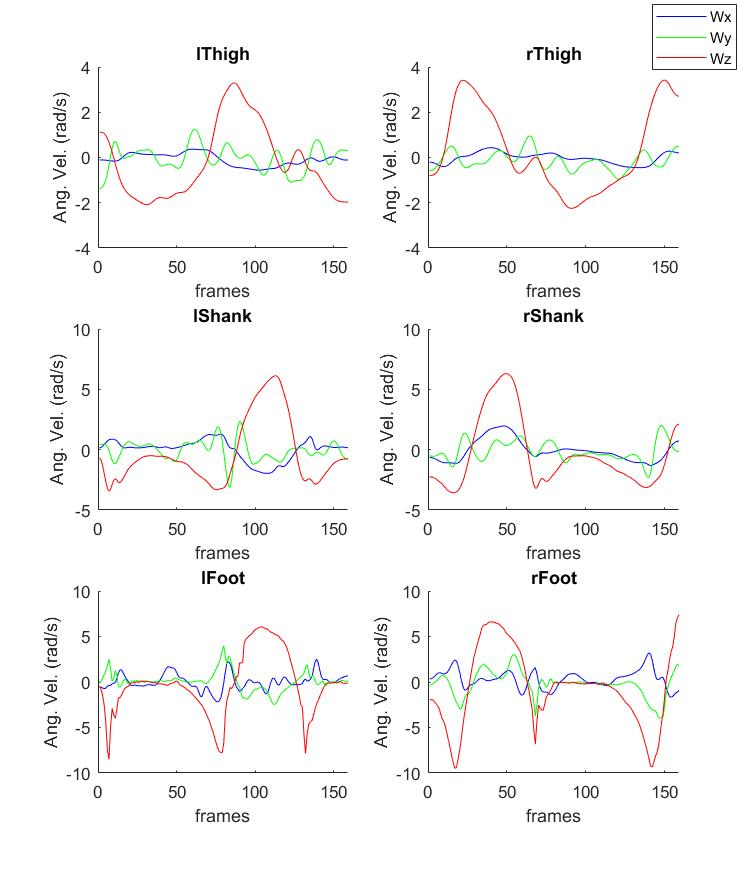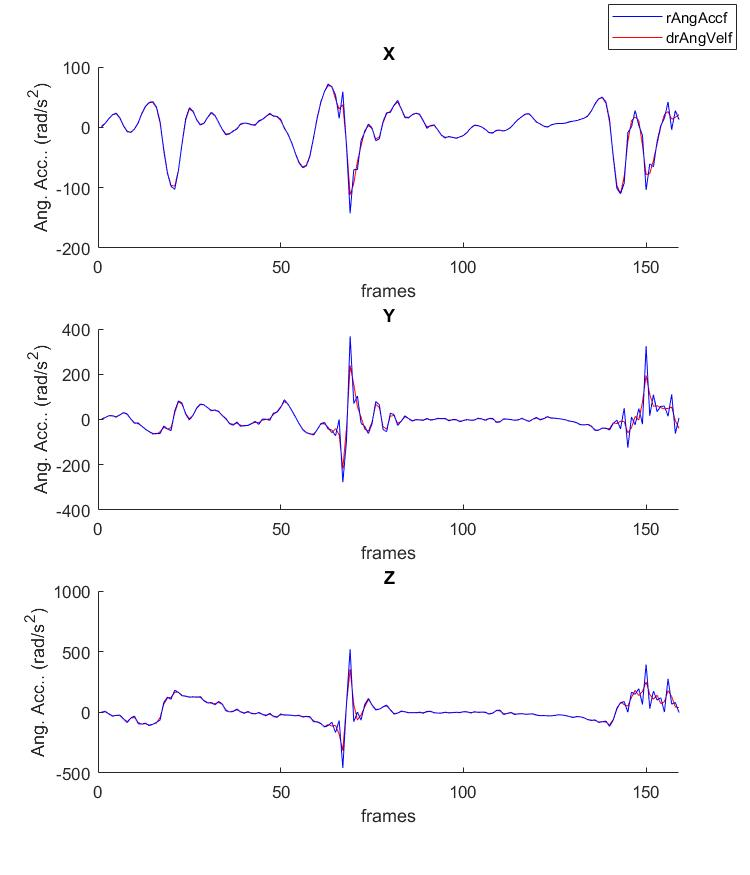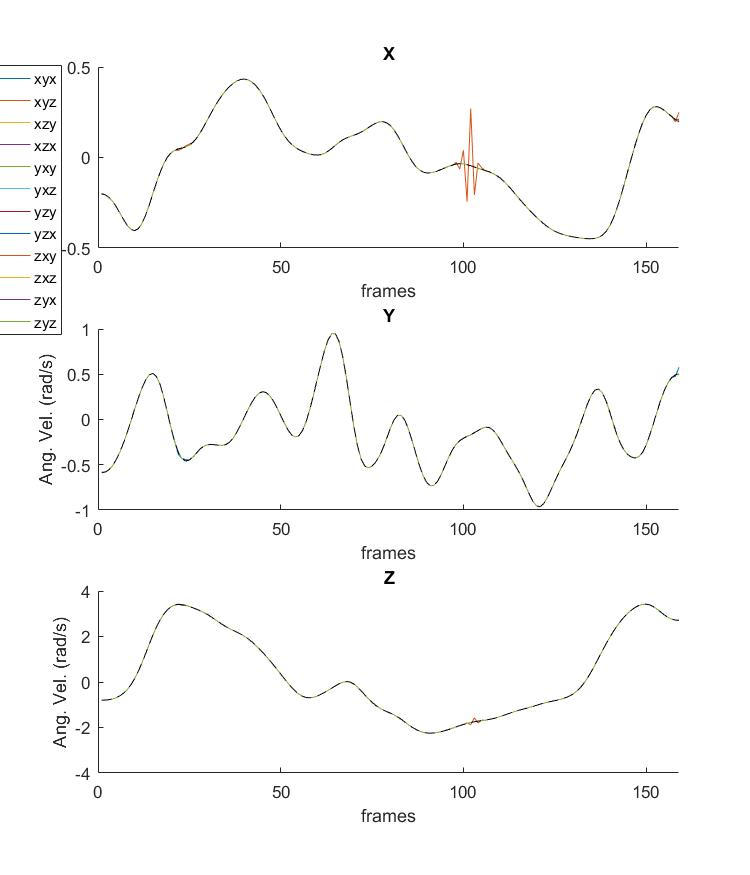### Week5: Quaternions (Euler Parameters)

1. Write the function "unwrapEP.m" to eliminate the discontinuity of Quaternions data.
2. Compare angular velocity & angular acceleration derived from Euler angle and Quaternions(EP).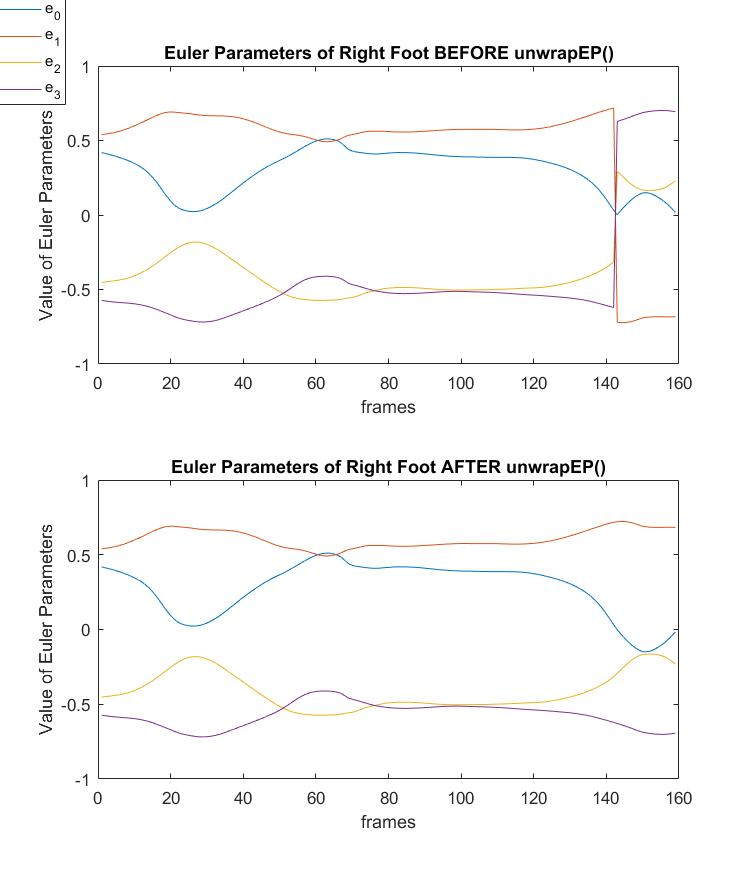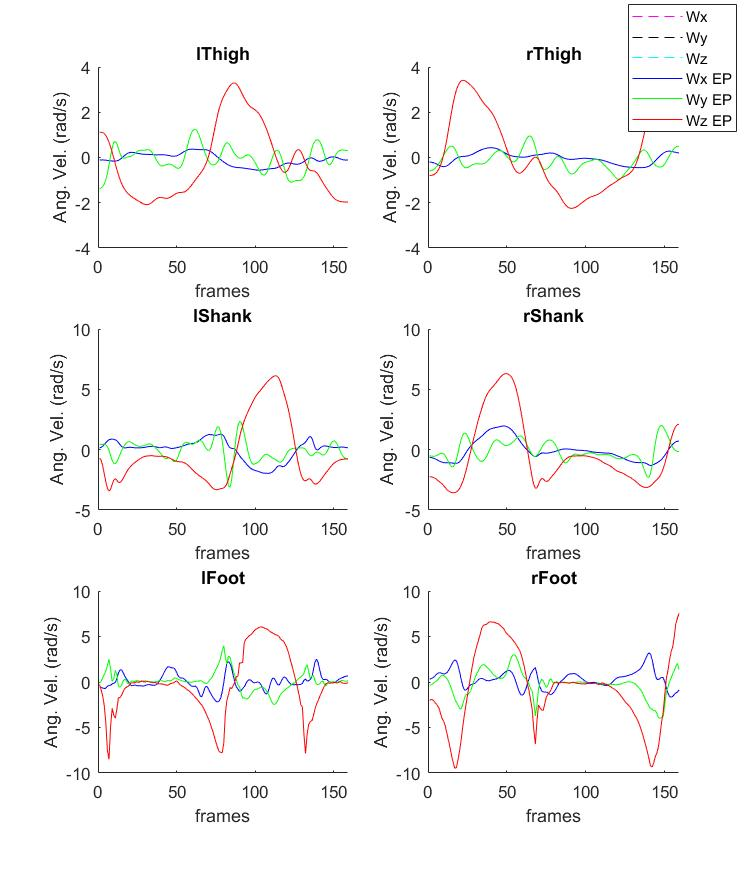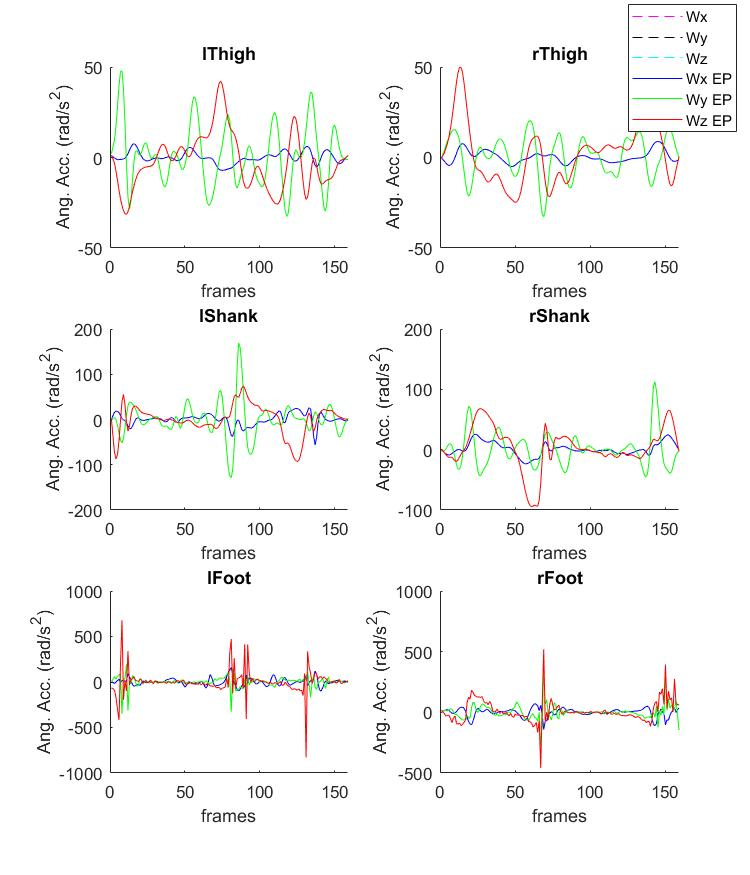### Week6: Screw Axis (Helical Axis)

1. Compare the rotation axis and angle derived from Screw axis and Quaternoins.
2. Determine the joint center & rotation axis with least-square error from several rotation axes dervied during the motion.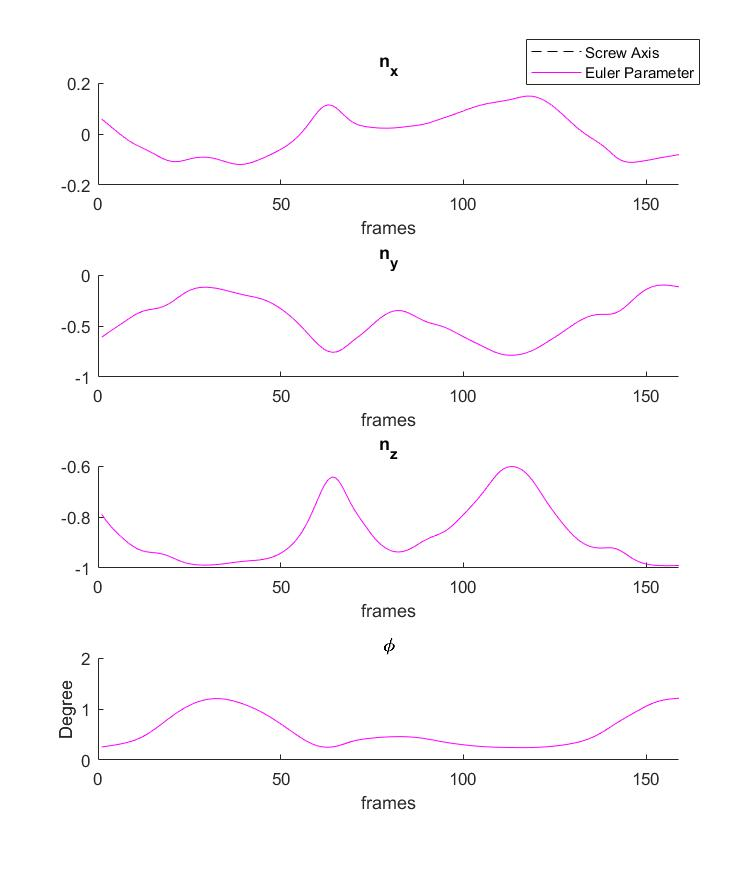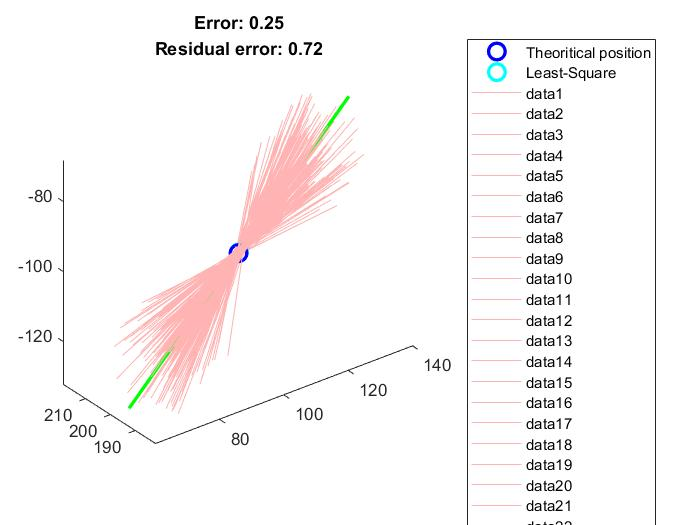### Week7: COM Tracking

1. Derive & compare the COM position of whole body using Dempster's anthropometrical data with simplifying the body model as 7, 11, 12, & 13 segments.
2. Display the COM positions. (Yellow: markers' position, green: COM of body segments, purple: COM of whole body).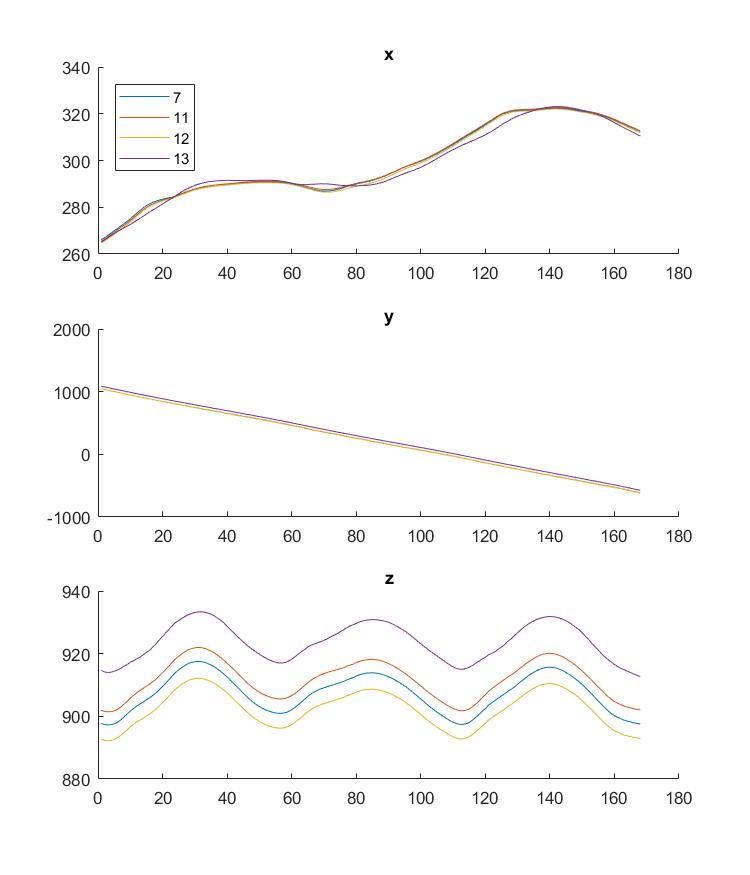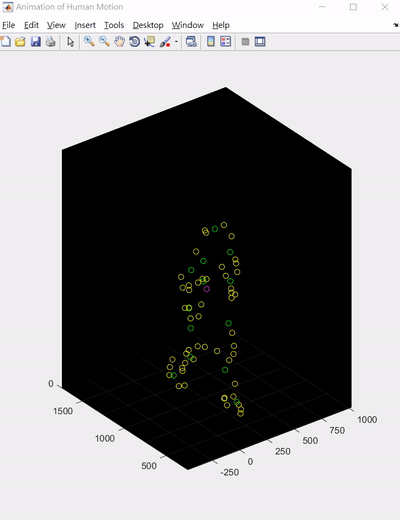### Week8: Evaluation of Body Balance

Derive the COP data during the recipient is standing still. Determined the eclipse covering 95% of these COPs with Principal COmponent Analysis (PCA). The area of the eclipse and length of its axes can be a indicator for evaluating one's body balancing ability.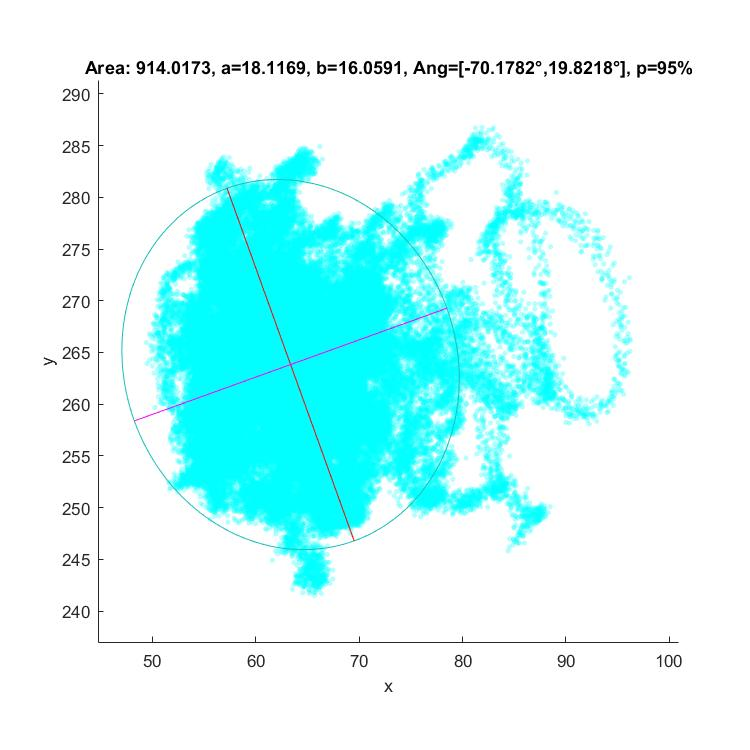### Week9: Angular Momentum

Derive the angular momentum and 1st derivation of angular momentum during the motion.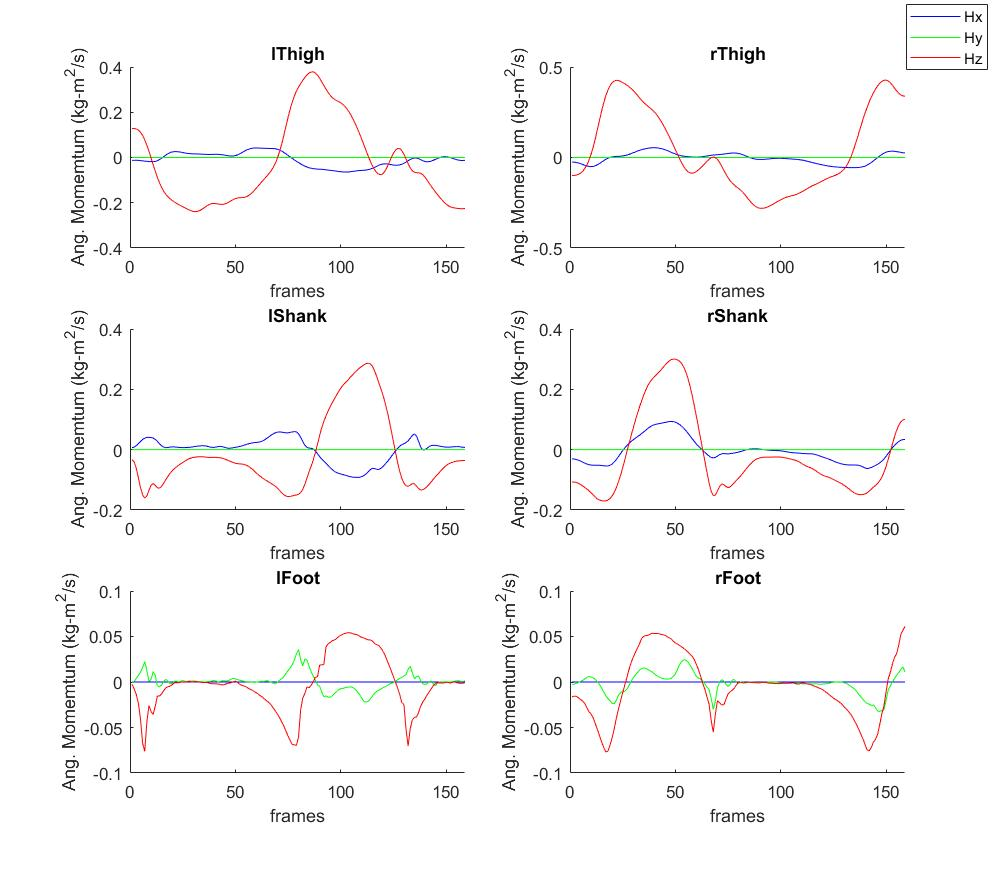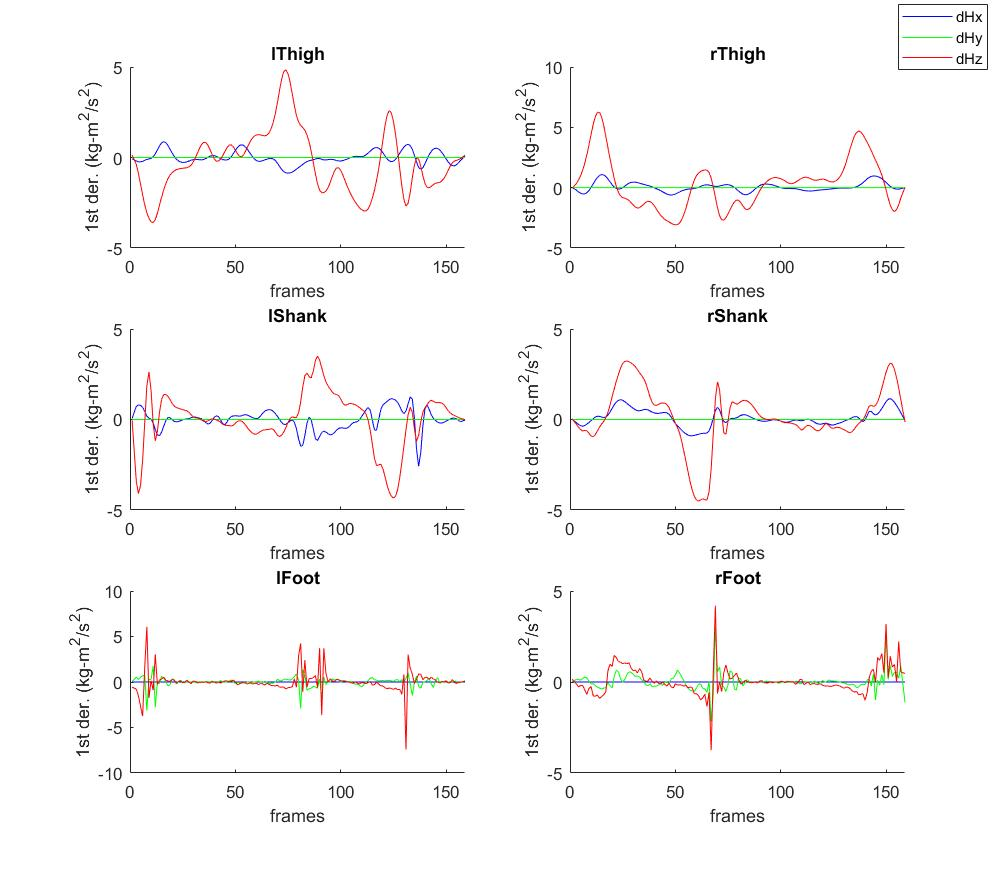### Week10: Joint Moment & Joint Force

Derive the joint moment & joint force of angular momentum during the motion. The results are normalized by being divided by the weight of recipient.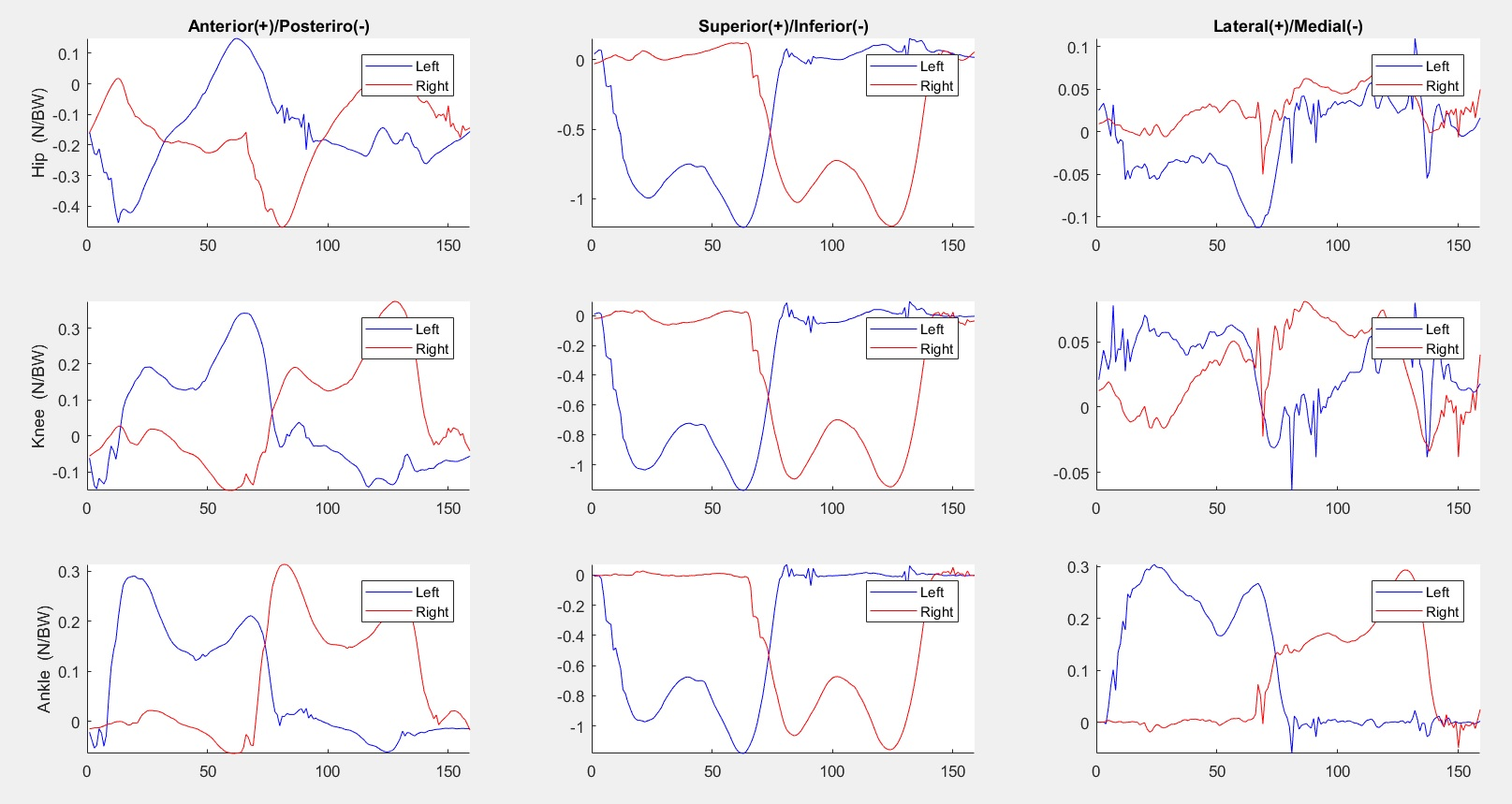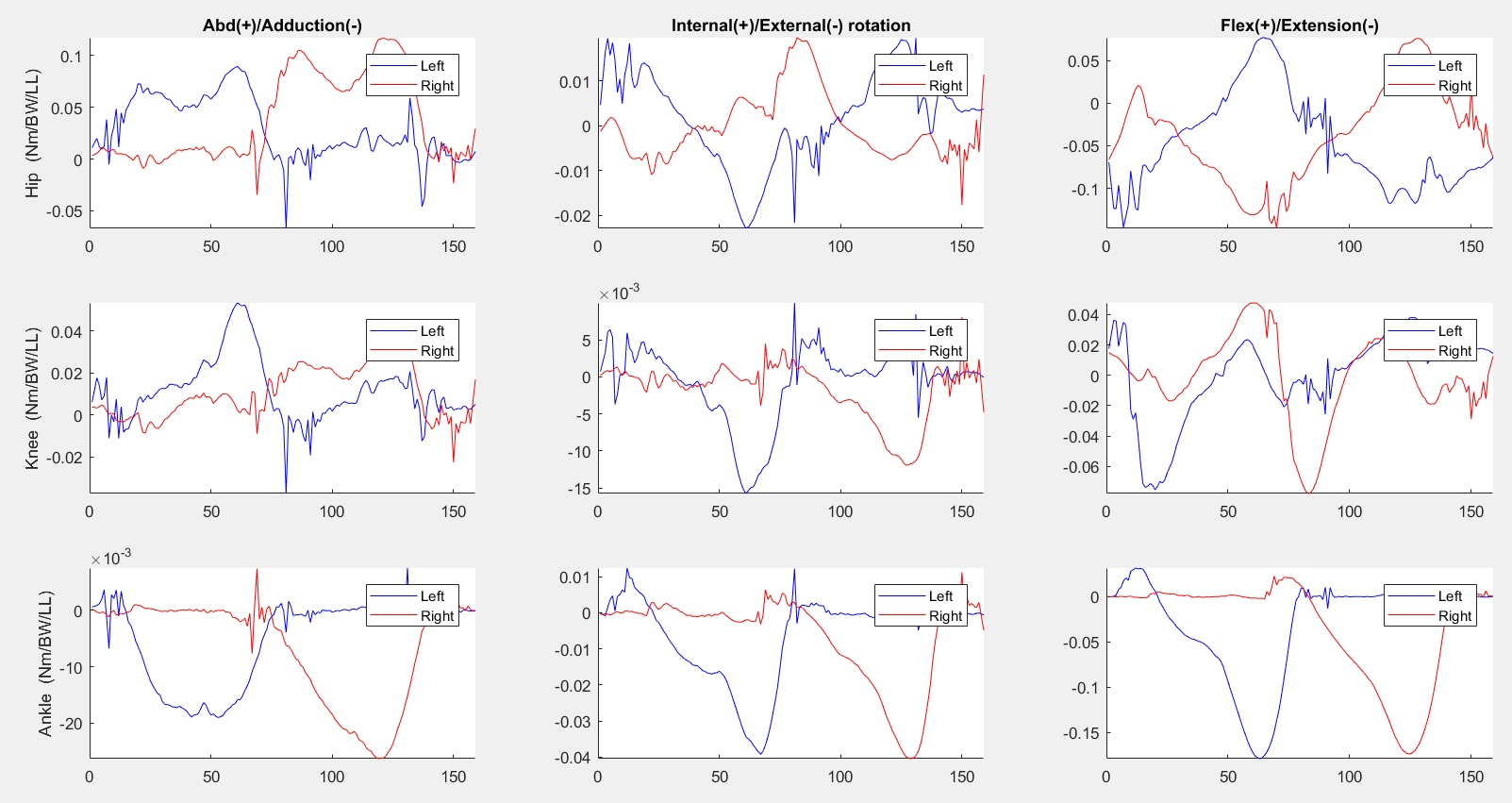## misc

In the last few minutes of Marvel's The Punisher S1E1 (Netflix), a similar result appeared when David Lieberman used gait detection and recognized Frank Castle, who faked dead after revenging for his family.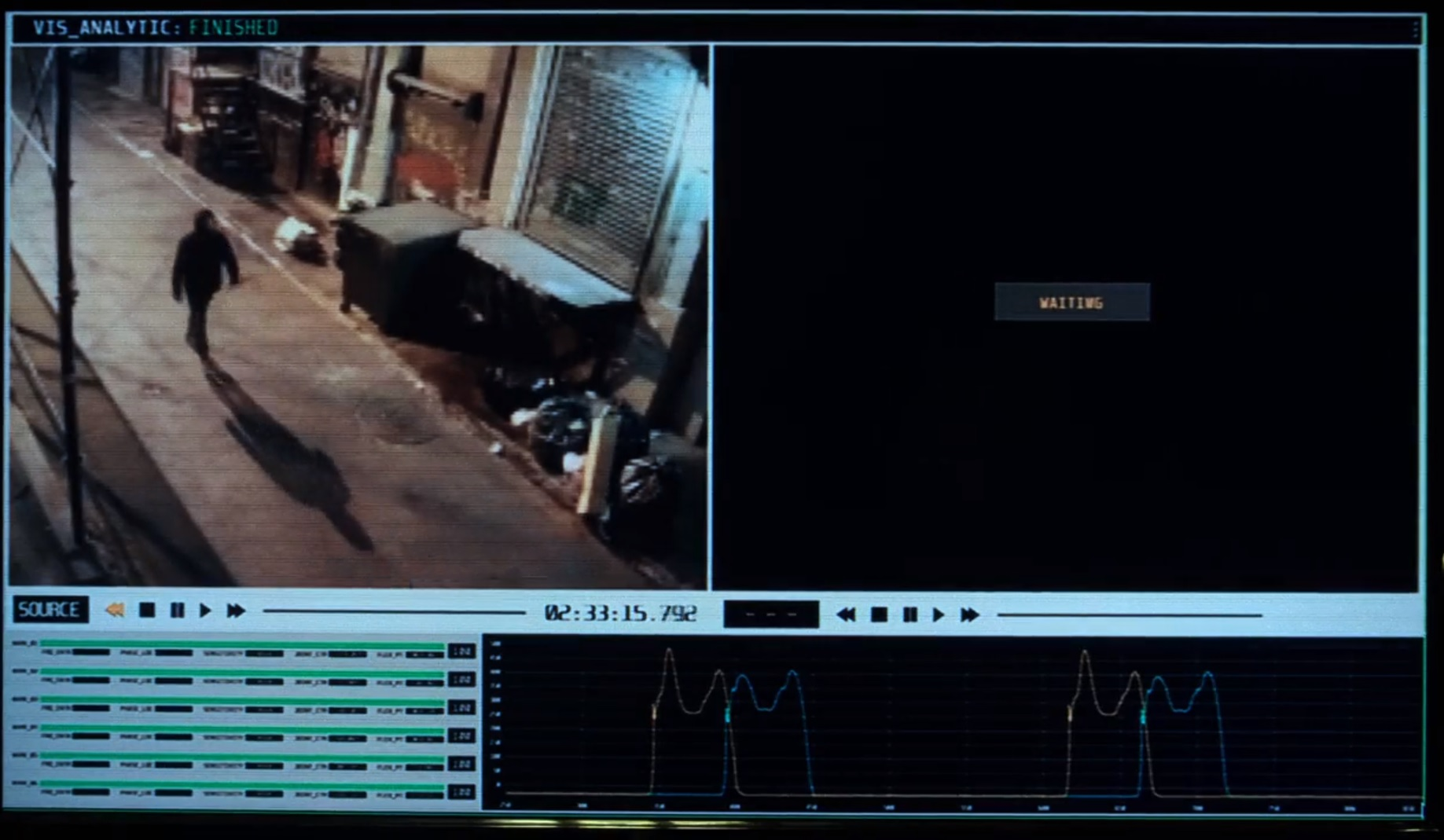### 인용 양식

Wei-Hsiang Wang (2022). Human-Motion-Analysis (https://github.com/mattwang44/Human-Motion-Analysis-MATLAB/releases/tag/0.0.1), GitHub. 검색됨 .

개발 환경: R2015b
모든 릴리스와 호환
##### 플랫폼 호환성
Windows macOS Linux

### Community Treasure Hunt

Find the treasures in MATLAB Central and discover how the community can help you!

Start Hunting!

#### final

이 GitHub 애드온의 문제를 보거나 보고하려면 GitHub 리포지토리로 가십시오.
이 GitHub 애드온의 문제를 보거나 보고하려면 GitHub 리포지토리로 가십시오.